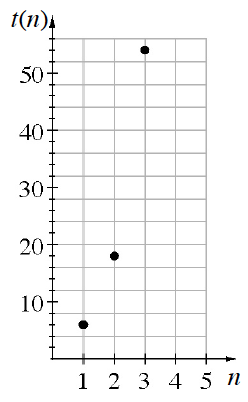### Home > CCA2 > Chapter B > Lesson B.2.3 > ProblemB-108

B-108.

Write an explicit equation for the sequence graphed at right.

Is this exponential or linear?

By what factor do the $y$-values increase as you look at the points from left to right?

$t(n) = 2(3)^n$